Jr Math section of MathBitsNotebook.comRadicals & IrrationalsTopics Standards: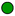NGMS-6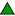CCSS 6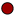NGMS-7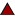CCSS 7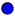NGMS-8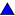CCSS 8

Squares, Cubes, Roots (intro: perfect squares & cubes = areas/volume, roots)Estimating Square Roots (2 methods, Square Root Algorithm)Radicals and Equations (equations of the form x2 = p and x3 = p)Irrational Numbers (definition, measurement, number line approximations, π )PRACTICE: • Practice with Radicals• Practice with Radicals and Equations• Practice with Irrationals• Practice with Simplifying Radicals Extra • Practice with Arithmetic of Radicals Extra • Vocabulary - Word Guess Game (topical vocabulary game - iPad compatible)